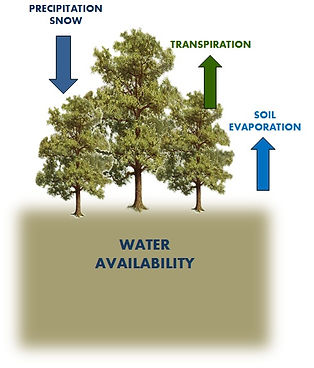## Water balance

The 3D-CMCC-FEM considers the soil as one bucket layer in which the water balance is computed on a daily time step considering inputs and outputs. Incoming water flux is constituted by daily precipitation, whereas water leaves the soil through total evapotranspiration, represented by canopy interception and transpiration, as well as soil evaporation.

The only input of water into the system occurs through precipitation either as rain or snow. Daily precipitation is also one of the required daily meteorological input variables. This precipitation is then routed to several potential pools.

The first resting place for incoming precipitation is the canopy intercepted rainwater pool. The amount of intercepted rainwater is a function of a user defined canopy interception coefficient, the amount of rainwater, and the Leaf Area Index (LAI, a unitless value that is the area of all leaves per unit ground area, m2 m-2).

Snow accumulates in a snow water pool when the temperature is below freezing and melts when the temperature is warmer than freezing. Snow also can sublimate when the temperature is less than freezing based on the amount of incoming solar radiation it receives.

If there is more than enough water to fill the canopy interception pool, the remaining water enters the soil water pool. The current soil water matric potential (MPa) is a function of the water in the soil now in relation to the soil’s saturated water holding capacity. Saturated soil water and field capacity soil water holding is defined based on the soil texture and depth (as specified in the site initialization file – percentage sand, silt, clay, and depth). The current soil water matric potential ( ψ soil ) is then determined by removing the calculated evaporation from the soil and the addition of water to the soil pool from precipitation (and snowmelt if there is any).

All evaporative processes (Canopy evaporation of intercepted water, transpiration during photosynthesis, and soil evaporation) are calculated using a modified Penman-Monteith Equation – PME. This equation calculates an evaporation rate that is a function of incoming radiation, vapor pressure deficit (VPD), and the conductances associated with the evaporation surface.

In the growing season, soil water availability (ASW) is computed for each canopy layer as:DeltaASW is given by the following formula in the growing season:and it’s calculated as: